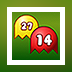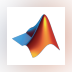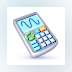### Math Man

Math Man is a pac man game with a twist. In this game you need to control the Math Man to eat all the ghosts.### OpenOffice.org

OpenOffice.org is an office suite designed for word processing, spreadsheets, presentations, graphics and databases.### MathType

MathType is an interactive equation editor that lets you create mathematical notations for word processing ...### MATLAB R2012a

MATLAB is a programming environment for algorithm development, data analysis, visualization, and numerical computation.### GeoGebra

GeoGebra is a mathematical tool that can solve equations, graph functions, create constructions, analyze data, and create 3D plots.### MiKTeX

MiKTeX (pronounced mick-tech) is an up-to-date implementation of TeX and related programs for Windows (all current variants).### Microsoft Mathematics

Microsoft Mathematics provides a set of mathematical tools that help students get school work done quickly and easily.

... calculator. Additional math tools help ...### QM for Windows

QM for Windows provides mathematical analysis for Operations Management, Quantitative methods, or Management Science.

... , Statistical functions, Game Theory, Goal ...### Graph

Graph is an open source application that can be used to draw mathematical graphs in a coordinate system.### Surfer